# C Program to Insertion Sort Using Array

In this tutorial you will learn about the C Program to Insertion Sort Using Array and its application with practical example.

## C Program to implement Insertion sort Algorithm

In this tutorial, we will learn to create a C program that will do insertion sort using C programming.

## Prerequisites

Before starting with this tutorial we assume that you are best aware of the following C programming topics:

• Operators in C Programming.
• Basic Input and Output function in C Programming.
• Basic C programming.
• For loop in C Programming.
• Creating and Using the user-defined function in C programming.

## What is Insertion Sort?

In every programming language, the sorting of data is a very important factor.  In C Language. Many different techniques for sorting are available to work with but in this tutorial, we will focus on the Insertion Sort Technique.

In this technique, the work is done by inserting the smaller number to the first position. i.e.

the first and second number is sorted if the number is big then it is moved to the right and if it is small the moves to the left.

## Output:-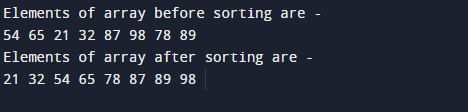In the above program, we have first declared and initialized a set of variables required in the program.

• n = it will hold the size of the array.
• x[]= it will hold the elements in an array.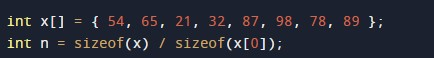Function to print array:-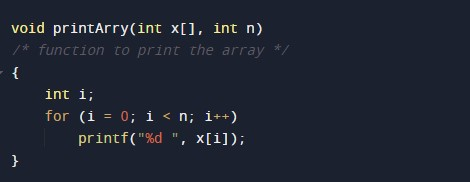Insertion sort function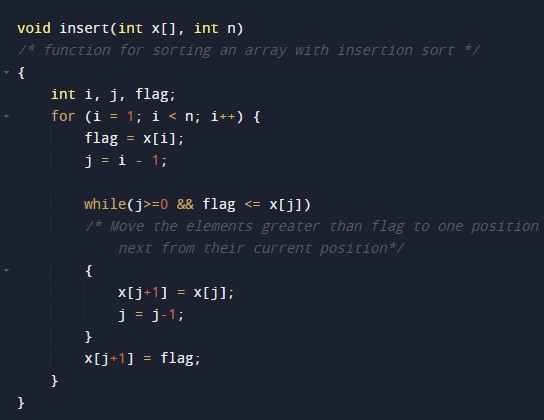Main function body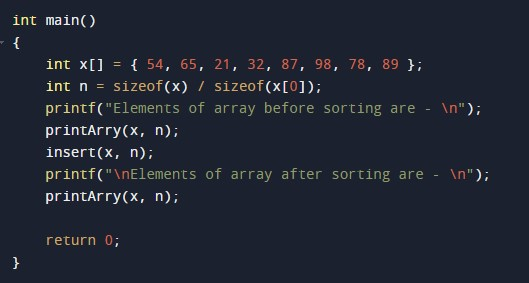In this tutorial we have learn about the C Program to Insertion Sort Using Array and its application with practical example. I hope you will like this tutorial.